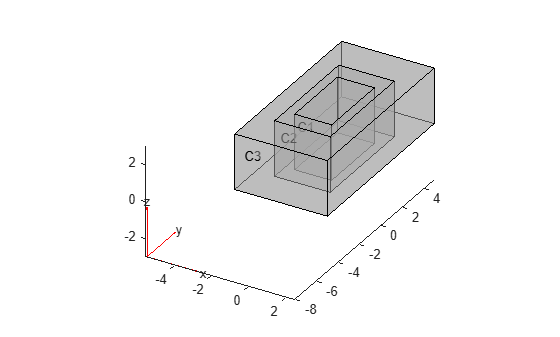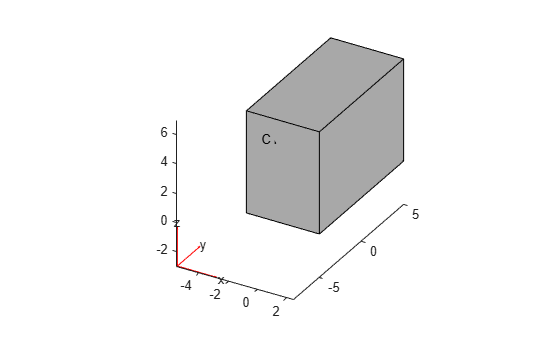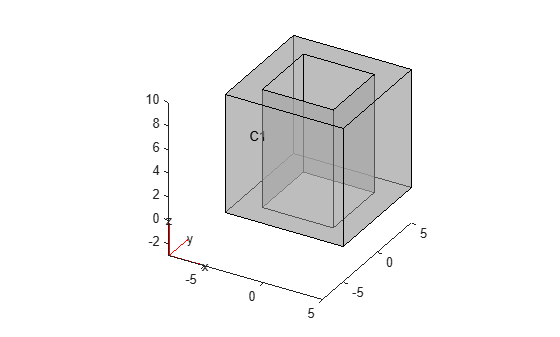# multicuboid

Create geometry formed by several cubic cells

## Syntax

``gm = multicuboid(W,D,H)``
``gm = multicuboid(W,D,H,Name,Value)``

## Description

example

````gm = multicuboid(W,D,H)` creates a geometry by combining several cubic cells.When creating each cuboid, `multicuboid` uses the following coordinate system.```

example

````gm = multicuboid(W,D,H,Name,Value)` creates a multi-cuboid geometry using one or more `Name,Value` pair arguments.```

## Examples

collapse all

Create a geometry that consists of three nested cuboids of the same height and include this geometry in a PDE model.

Create the geometry by using the `multicuboid` function. The resulting geometry consists of three cells.

`gm = multicuboid([2 3 5],[4 6 10],3)`
```gm = DiscreteGeometry with properties: NumCells: 3 NumFaces: 18 NumEdges: 36 NumVertices: 24 Vertices: [24x3 double] ```

Create a PDE model.

`model = createpde`
```model = PDEModel with properties: PDESystemSize: 1 IsTimeDependent: 0 Geometry: [] EquationCoefficients: [] BoundaryConditions: [] InitialConditions: [] Mesh: [] SolverOptions: [1x1 pde.PDESolverOptions] ```

Include the geometry in the model.

`model.Geometry = gm`
```model = PDEModel with properties: PDESystemSize: 1 IsTimeDependent: 0 Geometry: [1x1 DiscreteGeometry] EquationCoefficients: [] BoundaryConditions: [] InitialConditions: [] Mesh: [] SolverOptions: [1x1 pde.PDESolverOptions] ```

Plot the geometry.

`pdegplot(model,'CellLabels','on','FaceAlpha',0.5)`Create a geometry that consists of four stacked cuboids and include this geometry in a PDE model.

Create the geometry by using the `multicuboid` function with the `ZOffset` argument. The resulting geometry consists of four cells stacked on top of each other.

`gm = multicuboid(5,10,[1 2 3 4],'ZOffset',[0 1 3 6])`
```gm = DiscreteGeometry with properties: NumCells: 4 NumFaces: 21 NumEdges: 36 NumVertices: 20 Vertices: [20x3 double] ```

Create a PDE model.

`model = createpde`
```model = PDEModel with properties: PDESystemSize: 1 IsTimeDependent: 0 Geometry: [] EquationCoefficients: [] BoundaryConditions: [] InitialConditions: [] Mesh: [] SolverOptions: [1x1 pde.PDESolverOptions] ```

Include the geometry in the model.

`model.Geometry = gm`
```model = PDEModel with properties: PDESystemSize: 1 IsTimeDependent: 0 Geometry: [1x1 DiscreteGeometry] EquationCoefficients: [] BoundaryConditions: [] InitialConditions: [] Mesh: [] SolverOptions: [1x1 pde.PDESolverOptions] ```

Plot the geometry.

`pdegplot(model,'CellLabels','on','FaceAlpha',0.5)`Create a geometry that consists of a single cuboid and include this geometry in a PDE model.

Use the `multicuboid` function to create a single cuboid. The resulting geometry consists of one cell.

`gm = multicuboid(5,10,7)`
```gm = DiscreteGeometry with properties: NumCells: 1 NumFaces: 6 NumEdges: 12 NumVertices: 8 Vertices: [8x3 double] ```

Create a PDE model.

`model = createpde`
```model = PDEModel with properties: PDESystemSize: 1 IsTimeDependent: 0 Geometry: [] EquationCoefficients: [] BoundaryConditions: [] InitialConditions: [] Mesh: [] SolverOptions: [1x1 pde.PDESolverOptions] ```

Include the geometry in the model.

`model.Geometry = gm`
```model = PDEModel with properties: PDESystemSize: 1 IsTimeDependent: 0 Geometry: [1x1 DiscreteGeometry] EquationCoefficients: [] BoundaryConditions: [] InitialConditions: [] Mesh: [] SolverOptions: [1x1 pde.PDESolverOptions] ```

Plot the geometry.

`pdegplot(model,'CellLabels','on')`Create a hollow cube and include it as a geometry in a PDE model.

Create a hollow cube by using the `multicuboid` function with the `Void` argument. The resulting geometry consists of one cell.

`gm = multicuboid([6 10],[6 10],10,'Void',[true,false])`
```gm = DiscreteGeometry with properties: NumCells: 1 NumFaces: 10 NumEdges: 24 NumVertices: 16 Vertices: [16x3 double] ```

Create a PDE model.

`model = createpde`
```model = PDEModel with properties: PDESystemSize: 1 IsTimeDependent: 0 Geometry: [] EquationCoefficients: [] BoundaryConditions: [] InitialConditions: [] Mesh: [] SolverOptions: [1x1 pde.PDESolverOptions] ```

Include the geometry in the model.

`model.Geometry = gm`
```model = PDEModel with properties: PDESystemSize: 1 IsTimeDependent: 0 Geometry: [1x1 DiscreteGeometry] EquationCoefficients: [] BoundaryConditions: [] InitialConditions: [] Mesh: [] SolverOptions: [1x1 pde.PDESolverOptions] ```

Plot the geometry.

`pdegplot(model,'CellLabels','on','FaceAlpha',0.5)`## Input Arguments

collapse all

Cell width, specified as a positive real number or a vector of positive real numbers. If `W` is a vector, then `W(i)` specifies the width of the `i`th cell.

Width `W`, depth `D`, and height `H` can be scalars or vectors of the same length. For a combination of scalar and vector inputs, `multicuboid` replicates the scalar arguments into vectors of the same length.

Note

All cells in the geometry either must have the same height, or must have both the same width and the same depth.

Example: `gm = multicuboid([1 2 3],[2.5 4 5.5],5)`

Cell depth, specified as a positive real number or a vector of positive real numbers. If `D` is a vector, then `D(i)` specifies the depth of the `i`th cell.

Width `W`, depth `D`, and height `H` can be scalars or vectors of the same length. For a combination of scalar and vector inputs, `multicuboid` replicates the scalar arguments into vectors of the same length.

Note

All cells in the geometry either must have the same height, or must have both the same width and the same depth.

Example: `gm = multicuboid([1 2 3],[2.5 4 5.5],5)`

Cell height, specified as a positive real number or a vector of positive real numbers. If `H` is a vector, then `H(i)` specifies the height of the `i`th cell.

Width `W`, depth `D`, and height `H` can be scalars or vectors of the same length. For a combination of scalar and vector inputs, `multicuboid` replicates the scalar arguments into vectors of the same length.

Note

All cells in the geometry either must have the same height, or must have both the same width and the same depth.

Example: ```gm = multicuboid(4,5,[1 2 3],'ZOffset',[0 1 3])```

### Name-Value Arguments

Specify optional pairs of arguments as `Name1=Value1,...,NameN=ValueN`, where `Name` is the argument name and `Value` is the corresponding value. Name-value arguments must appear after other arguments, but the order of the pairs does not matter.

Before R2021a, use commas to separate each name and value, and enclose `Name` in quotes.

Example: `gm = multicuboid([1 2],[1 2],[3 3],'Void',[true,false])`

Z offset for each cell, specified as a vector of real numbers. `ZOffset(i)` specifies the Z offset of the `i`th cell. This vector must have the same length as the width vector `W`, depth vector `D`, or height vector `H`.

Note

The `ZOffset` argument is valid only if the width and depth are constant for all cells in the geometry.

Example: ```gm = multicuboid(20,30,[10 10],'ZOffset',[0 10])```

Data Types: `double`

Empty cell indicator, specified as a vector of logical `true` or `false` values. This vector must have the same length as the width vector `W`, depth vector `D`, or the height vector `H`.

The value `true` corresponds to an empty cell. By default, `multicuboid` assumes that all cells are not empty.

Example: `gm = multicuboid([1 2],[1 2],[3 3],'Void',[true,false])`

Data Types: `double`

## Output Arguments

collapse all

Geometry object, returned as a DiscreteGeometry Properties object.

## Limitations

• `multicuboid` lets you create only geometries consisting of stacked or nested cuboids. For nested cuboids, the height must be the same for all cells in the geometry. For stacked cuboids, the width and depth must be the same for all cells in the geometry. Use the `ZOffset` argument to stack the cells on top of each other without overlapping them.

• `multicuboid` does not let you create nested cuboids of the same width and depth. The call `multicuboid(w,d,[h1,h2,...])` is not supported.

## Version History

Introduced in R2017a